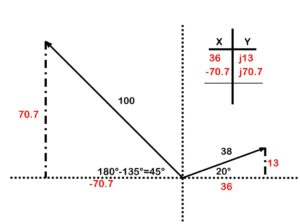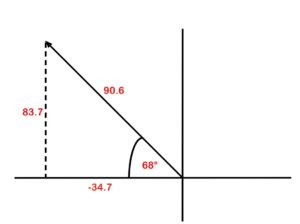## A quick recap

There was a fair bit to wrap our heads around before we finally got into vector addition. Here are some of the key points:

• Vectors contain magnitude (resultant) and direction (angle).
• Each vector can be broken into X and Y coordinates.
• We must use a quadrant system to chart the X and Y coordinates.
• Pay attention to the polarity (what quadrant is it in?).
• Vectors can be expressed in the polar form (resultant and angle) or rectangular form (X and Y coordinates).
• Base your angle off of the X axis.
• When converting from rectangular to polar, it is extremely important to pay attention to what quadrant you are in.
• Quadrant 1 is the angle you calculate.
• Quadrant 2 is 180 minus the angle you calculate.
• Quadrant 3 is 180 plus the angle you calculate.
• Quadrant 4 is either 360 minus the angle you calculate, or, put a negative in front of the angle you calculate.

Okay, let’s learn how to add vectors.

When adding vectors, we have to find some common ground. This is why we focus on the X and Y coordinates. Each vector can be broken down into X and Y coordinates. This allows us to find some common ground as the X coordinates are heading in the same direction and the Y coordinates are heading in the same direction. Let’s look at the example in Figure 39.

In this example, we have one vector that is 38 V @ 20 degrees and another that is 100 V @ 135 degrees.The first step is to draw in the X and Y axes. (See Figure 40.) This will help provide us with a reference when determining our X and Y coordinates.Next we will work out the X and the Y for each vector, in Figure 41.Figure 41. Adding vectors determining X and Y

Next add an X-Y chart and enter the coordinates (Figure 42).Figure 42. Adding vectors X and Y chartFigure 43. Adding vectors for rectangular form

Take your rectangular form and chart it on its own (Figure 44).Figure 44. Adding vectors for polar form

Take the resultant and the angle, and convert it to polar form: 90.6 @ (180°−68°)90.6 @ 112°.

There you have it. If you have more vectors, you just keep adding other rows to your X-Y chart.

Video! This video walks through how to add vectors heading in completely different directions.